Next: Homenergic Homentropic Flow Up: Two-Dimensional Compressible Inviscid Flow Previous: Thin-Airfoil Theory

# Crocco's Theorem

Bernoulli's theorem for the steady, inviscid flow of an ideal gas, in the absence of body forces, implies that, on a given streamline,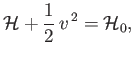(15.85)

where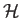is the specific enthalpy,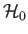the stagnation enthalpy, and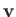the flow velocity. (See Section 14.5.) The fluid equation of motion, (14.31), reduces to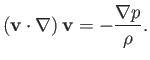(15.86)

However, according to Equation (A.171),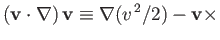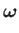(15.87)

where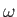. Hence, we obtain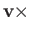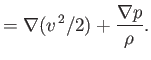(15.88)

Now, Equation (14.29) implies that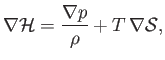(15.89)

whereis the temperature, and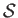the specific entropy. Moreover, Equation (15.85) yields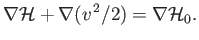(15.90)

Thus, we arrive at Crocco's theorem: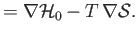(15.91)

In most aerodynamic flows, the fluid originates from a common reservoir, which implies that the stagnation enthalpy,, is the same on all streamlines. Such flow is termed homenergic. It follows from Equation (14.18) that the stagnation temperature,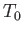, is the same on all streamlines in homenergic flow. According to Crocco's theorem, an irrotational (i.e.,everywhere) homenergic (i.e.,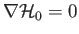everywhere) flow pattern is also homentropic (i.e.,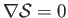everywhere). Conversely, a homenergic, homentropic flow pattern is also irrotational (at least, in two dimensions, whereandcannot be parallel to one another).Next: Homenergic Homentropic Flow Up: Two-Dimensional Compressible Inviscid Flow Previous: Thin-Airfoil Theory
Richard Fitzpatrick 2016-03-31